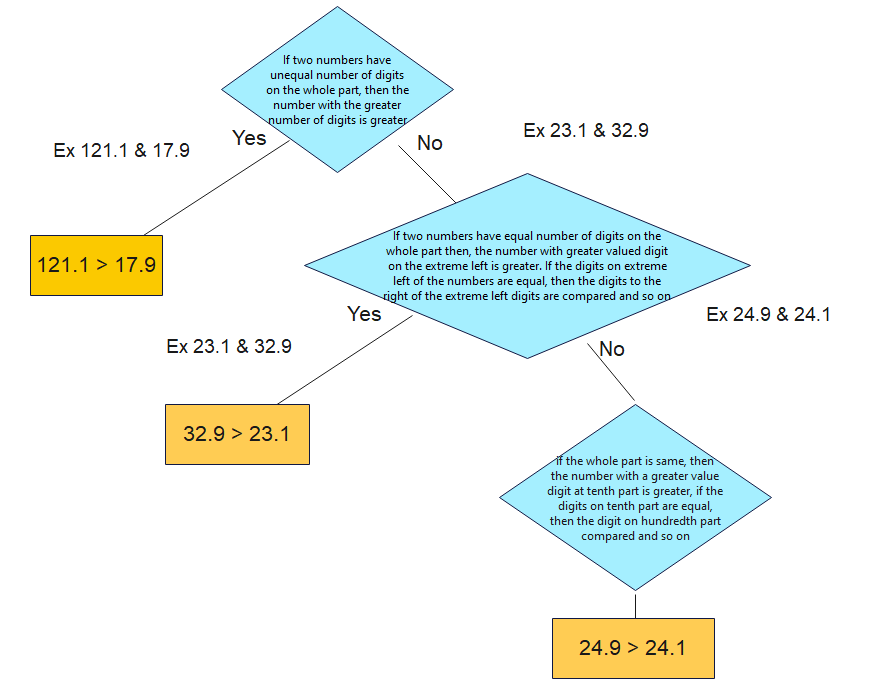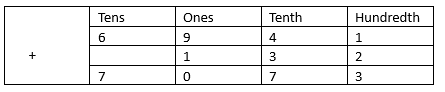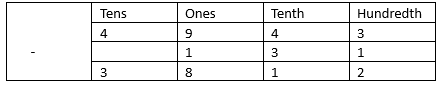# Notes for Class 6 Maths Decimals

In this page we have Notes for Class 6 Maths Decimals . Hope you like them and do not forget to like , social share and comment at the end of the page.
Tenths and Hundred is deleted as per Rationalized Syllabus, so you may ignore questions on them

## Decimal Number

A decimal number is a number that uses a decimal point to separate the whole number part from the fractional part. For example, 12.34 has a whole number part of 12 and a fractional part of 34/100.

## Decimal Point

The decimal point is a symbol used to separate the whole number from the fractional part in a decimal number.

## Place Value

In a decimal number, each digit has a place value based on its position relative to the decimal point. For example, in 12.34, the digit 1 is in the 'tens' place, and the digit 4 is in the 'hundredths' place.

## Comparing the Decimals

To find larger number, we can follow these steps
(1) When comparing two positive decimals, here are the tips
(a) If two numbers have unequal number of digits on the whole part, then the number with the greater number of digits is greater.
(b) If two numbers have equal number of digits on the whole part then, the number with greater valued digit on the extreme left is greater. If the digits on extreme left of the numbers are equal, then the digits to the right of the extreme left digits are compared and so on.
(c) if the whole part is same, then the number with a greater value digit at tenth part is greater, if the digits on tenth part are equal, then the digit on hundredth part compared and so on(2) When comparing two negative decimals, we can remove the sign and compare them as positive integer given above, the smallest of them would be the greater when we put the sign back
(3)We comparing two different sign decimals, the number with positive sign is larger

## Length

100 cm =1 m
1 cm = (1/100) m
Therefore
45 cm = 45/100 m = .45 m

## Weigth

1000 g =1 kg
1 g = (1/1000) kg
Therefore
532 g = 532/1000 kg = .532 kg

Addition of decimals can be done by adding hundredths from hundredths, tenths from tenths, ones from ones and so on
This we can add decimals in the same way as whole numbers
Example69.41 + 1.32=70.73
Another one
12.34+5.67=18.01

## Subtraction of Decimals

Subtraction of decimals can be done by subtracting hundredths from hundredths, tenths from tenths, ones from ones and so on
This we can subtract decimals in the same way as whole numbers
Example49.43 - 1.31=38.12
Another Example
12.34−5.67=6.67### Practice Question

Question 1 What is $\frac {1}{2} + \frac {3}{4}$ ?
A)$\frac {5}{4}$
B)$\frac {1}{4}$
C)$1$
D)$\frac {4}{5}$
Question 2 Pinhole camera produces an ?
A)An erect and small image
B)an Inverted and small image
C)An inverted and enlarged image
D)None of the above